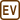Diameter Increase of Cylinder When Being Heated

Is it possible to use a micrometer with accuracy of 0.01 mm to measure the increase of diameter of a brass cylinder caused by heating the cylinder from a temperature of 5 °C to 35 °C?

At the temperature of 5 °C the diameter of the cylinder is 20.45 mm.

• Hint

What relationship must apply between the measured quantity and the accuracy of the measurement device, so that we can use the device to measure the quantity?

• Analysis

To measure the increase of the diameter of the cylinder using the given micrometer, the change of the diameter must be greater than the accuracy of the micrometer.

The change in diameter the cylinder can be determined using the formula for thermal linear expansion.

• Given values

 t0 = 5 °C initial temperature of the cylinder t1 = 35 °C final temperature of the cylinder d0 = 20.45 mm diameter of the cylinder at initial temperature Δd = ? change in diameter of the cylinder

From The Handbook of Chemistry and Physics:

 α = 18.7·10−6 °C−1 coefficient of thermal expansion of brass
• Solution

We must first determine the change in diameter Δd which is caused by heating of the cylinder. We use the well-known formula for linear expansion.

It is true that:

Δd = d0αΔt = d0α(t1t0),

where d0 is the initial diameter of the cylinder, t0 the initial temperature, t1 the final temperature, and α is the coefficient of thermal expansion of brass.

• Numerical solution

$\mathrm{\Delta} d=d_{0}\alpha(t_{1}-t_{0})=20.45\cdot{18.7}\cdot{10^{-6}}\cdot{(35-5)}\, \mathrm{mm}$ $\mathrm{\Delta} d\,\dot=\, 0.011\, \mathrm{mm}$